# QlikView App Dev

Discussion Board for collaboration related to QlikView App Development.

Announcements
Support Cases coming to Qlik Community Oct. 4! Start chats, open cases, explore resources: READ DETAILS
cancel
Showing results for
Did you mean:Not applicable

## count until value changes (in diagram)

hello and a happy new year to everyone

i've got a question about a counting function:

how can i count the rows until the value in one column changes.

ex:

A

A

A

A

A

A

B

B

B

A

A

A

A

i want to count all "A's" before the value changes to "B"

1 Solution

Accepted Solutions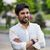MVP

Script:

Table:

If(Peek('Example') = Example, Peek('Same'), RangeSum(1, Peek('Same'))) as Same;

Example

A

A

A

A

B

B

B

B

A

A

B

B

B

];

Straight Table

Dimension: Example and Same

Expression: Count(Example)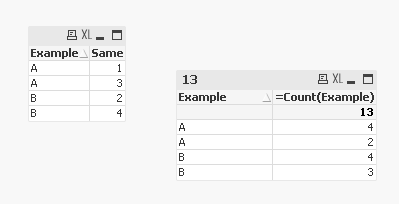10 RepliesMVP

Where are you wanting to count this? Script or front end? And you only want to count As? and not BsNot applicable
Author

i think i will count on diagram. but it can be in script too.

yes, i have to know how much rows are there before the first B is in. I want to count the Bs as well, but only the uninterrupted Bs.

Example

A

A

A

A

B

B

B

B

A

A

B

B

B

In this example i would like to count the first As, then the First Bs, then de second As and the second Bs - ist that even possible?MVP

Script:

Table:

If(Peek('Example') = Example, Peek('Same'), RangeSum(1, Peek('Same'))) as Same;

Example

A

A

A

A

B

B

B

B

A

A

B

B

B

];

Straight Table

Dimension: Example and Same

Expression: Count(Example)MVP

Hi,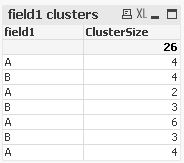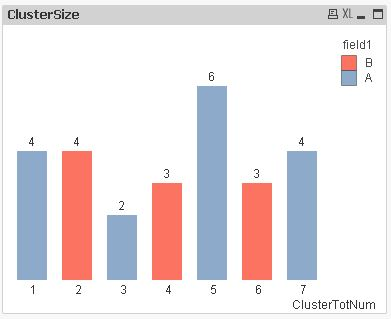```table1:
AutoNumber(ID,ClusterTotNum) as field1Seq,
AutoNumber(ClusterTotNum,field1) as ClusterNum;
*,
Alt(Peek(ClusterTotNum),0)-(Previous(field1)<>field1) as ClusterTotNum
Inline [
field1
A
A
A
A
B
B
B
B
A
A
B
B
B
A
A
A
A
A
A
B
B
B
A
A
A
A
];

Join
Count(field1) as ClusterSize
Resident table1
Group By ClusterTotNum;
```Not applicable
Author

thank you! this will help me!Not applicable
Author

thank you! this will help me!MVP

you're welcome.

If you like, you can mark this solution helpful.

thanks

regards

MarcoNot applicable
Author

what if I want to count the number of clusters with the same label, output example:

A: 4

B: 3

Thanks!Not applicable
Author

I figured it out by using the expression as max(ClusterNum):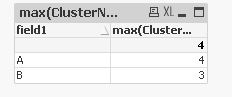This is very useful to count the number of clusters.Community Browser